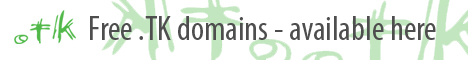.tk Renaming InternetMake sure you understand Rubik's Cube Notation.
Pictures courtesy of Amaury Sechet.

There are 57 OLL (orientation of the last layer) cases. Using these algorithms you can orient any given case in one step. Put simply, this step makes the top face one solid color. All knowledgeable cubers would recommend finishing learning all the PLLs before you begin tackling full OLL.

Thanks for the OLL Images, Lance.

R U R' U R U2 R'

R U2 R2 U' R2 U' R2 U2 R

F' (r U R' U') r' F R

( R U R' U') M’ U R U' r'

F (R U R' U') F'

R' (F R U R' U' F') U R

R' U' (R' F R F') U R

r’ U (r U r’ U’) r y R U’ R’

r U r' (R U R' U') r U' r'

R U2 R2 U' R U' R' U2 F R F'

F (R U R' U') R F' (r U R' U') r'

f (R U R' U') f'

R U B' U' R' U R B R'

(R U R' U) R U' R' U' (R' F R F')

r U R' U R U' R' U R U2 r'

F R U R' U' R U R' U' F'

R B’ R2 F R2 B R2 F’ R

F R U' R' U' R U R' F'

r U2 R' U' R U' r'

r U R' U R U2 r'

r R2 U’ R U’ R’ U2 R U’ r’ R

R U R' y R' F R U' R' F' R

(R' F R F') R U2 R' U’ (F’ U’ F)

R U R' U R U' R' U R U' R' U' (R' F R F')

M U (R U R' U') M2 (U R U' r')

M U (R U R’ U’) r R2 F R F’

(f R U R' U' f') U' (F R U R' U' F')

(F R U R' U' F') (f R U R' U' f')

R' U' R U' R' U2 R

R U2 R' U' R U R' U' R U' R'

(r U R' U') r' F R F'

M' U M U2 M' U M

(R U R' U') (R' F R F')

L (F' L' U' L U F) U' L'

(R U R' U') x D' R' U R U' D

l U' (l' U' l U) l' y L' U L

l’ U’ l (L’ U’ L U) l’ U l

R' U' R U' R' U y' R' U R B

F (U R U' R') (U R U' R') F'

F' (U' L' U L) F

R' U' (F U R U' R' F') R

(R' U' R U') R' U R U R B' R' B

r' U' R U' R' U R U' R' U2 r

R' U' l' U R U' R' U R U' x' U R

R' F R2 B' R2 F' R2 B R'

R U2 R2' F R F' R U2 R'

r' U2 R U R' U r

r' U' R U' R' U2 r

r' R2 U R' U R U2 R' U r R'

(R’ U’ R) y’ x’ (R U’ R’) F (R U R’)

R2' U R' B' R U' R2' U R B R'

(R’ F R F’) (R’ F R F’) (R U R’ U’) R U R’

R U R' U (R' F R F') U2 (R' F R F)'

R U2' R2' F R F' U2 M' U R U' r'

(f R U R' U' f') U (F R U R' U' F’)

R U2 (R2' F R F') U2' (R' F R F')

Katekyo Hitman Reborn